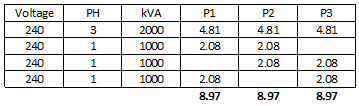# Single phase circuit on 3 phase current calc

 Hi,I have a question regarding calculating current in a 3 phase supply. I have my head around how to calculate the current in a 3 phase circuit. For example if we have a 22kw load assuming a power factor of 0.8 would be 22000/(1.732x415x0.8) = 38.25AHowever if I’m installing a 7.2kw single phase circuit on a 3-phase supply I.e using L1 (or L2 or L3) and neutral. Is the calc 7200/(1.732x230x0.8) = 18.06 ampsIt’s not the usual 7200/230 (simplified calc) where the current would be 31.30A we would use for a single phase supply?. Even though it is a single phase circuit?.Thanks,A. asked 6/15/2019 spark83192Hi Steven, I have a related question.   We often utilize 240V 3PH to supply mixed 3PH loads and 1PH 240V loads on any two phases. I want to confirm the calculation of current in the single phase loads at the line-to-line voltage in the following simplified example where the current for a single phase load of 1kVA = (1000VA / 2) / 240V = 2.08A (as opposed to 1000VA/240V = 4.17A).  The resulting system would then have a balanced 3PH load of approximately 9A.Thanks. answered 3 months ago tnhokie27 2edited 3 months ago tnhokie272Looks like in your first example you are using 415 V, and 230 in your second.  The common convention is 415/240 or 400/230 V  [line-line/line-neutral]. This is how I like to calculate: First example: 22000/0.8 = 27500 VA.  I =  (27500/3) / 240 = 38.2.  Same as your answer, just a slightly different way of calculating (and using 240 V, whereas 415/1.732 = 239.6 more accurately).   Second example: 7200/0.8 = 90000VA = I = 9000/230 = 39.1 A.   In your formulae using 1.732 x voltage assumes the voltage is line-line.  In the second example, the voltage is line-neutral.  Hope this helps. answered 6/16/2019 Steven McFadyen 246In order to provide an answer to this question, you must Login

## I am flagging this question because...

10 inform moderator flags remaining

## I am flagging this answer because...

10 inform moderator flags remaining

## Flagging a Post

You have already flagged this post. Clicking "Remove Flag" below will remove your flag, thus reducing the count by one as well.

## I am deleting this answer because...

Aluminium Windings - Dry Type Transformers

The other day I was talking to a colleague who is a building services consultant.  Despite regularly specifying dry-type/cast resin transformers he was...

Cables for MV Power Distribution - Earthed versus Unearthed Systems

Power cables can basically be classified into earthed and unearthed cables, where earthed and unearthed refer to the application for which the cable is...

Three phase systems are derived from three separate windings, either connected in delta or star (wye). Each winding can be treated separately, leading...

Periodic Electrical Installation Inspection – How Often?

How often installations are inspected is up to the owner of the installation, provided such durations do not exceed any regulatory maximums in force. ...

Maxwell's Equations - Introduction

Maxwell's Equations are a set of fundamental relationships, which govern how electric and magnetic fields interact. The equations explain how these fields...

Fault Calculation - Symmetrical Components

For unbalance conditions the calculation of fault currents is more complex. One method of dealing with this is symmetrical components. Using symmetrical...

Alternating Current Circuits

Alternating current (a.c.) is the backbone of modern electrical power distribution. In this article I’ll be pulling some of the more important concepts...

Maximum Demand for Buildings

Estimating maximum demand is a topic frequently discussed. Working out how much power to allow for a building can be very subjective . Allowing too much...

Understanding LV Circuit Breaker Fault Ratings

I think this post is going to be helpful to several of our readers. While the IEC low voltage circuit breaker Standard [IEC 60947-2, Low voltage switchgear...